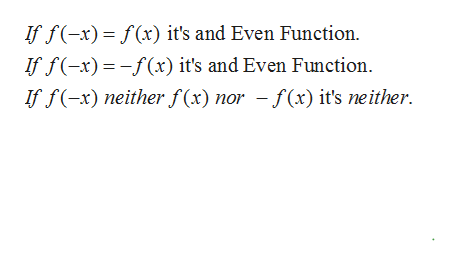# Determine whether the function is even, odd, or neither.f(x)=3x7+4x5

Question
13 views

Determine whether the function is even, odd, or neither.

f(x)=3x7+4x5

check_circle

Step 1

We can follow the rules of even and odd functions on the board.help_outlineImage TranscriptioncloseIf f(x) f(x) it's and Even Function. f fx) x) it's and Even Function If f(-x) neither f(x) nor - f(x) it's neither fullscreen
Step 2

Let us find f(-x) function by substituting x...

### Want to see the full answer?

See Solution

#### Want to see this answer and more?

Solutions are written by subject experts who are available 24/7. Questions are typically answered within 1 hour.*

See Solution
*Response times may vary by subject and question.
Tagged in

### Other